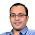## Nov 10, 2012

### Sam Loyd Puzzle Solvability

Source: 15 Puzzle

Problem: The 15-puzzle (also called Gem Puzzle, Boss Puzzle, Game of Fifteen, Mystic Square and many others) is a sliding puzzle that consists of a frame of numbered square tiles in random order with one tile missing. The object of the puzzle is to place the tiles in order (from figure at left to figure at right) by making sliding moves that use the empty space. Prove that the 15 puzzle in the configuration as shown on the left is not solvable.I made this fb game yesterday night - 9 Puzzle over Movie Posters - Movie Sliding Puzzle
Sam Loyd published some great puzzles in his time. One book that gets recommended a lot is "Sam Loyd's Cyclopedia of 5000 Puzzles tricks and Conundrums" (Amazon , Flipkart)

Update: (24/12/2012)
Solution provided by Raghuram Kowdeed (IIT Kanpur) in comments!

Update: (24/12/2012)
Same solution posted by Sai Teja Pratap (IIT Bombay CSE Final Year Student) and Eeshaan Malhotra (IIT Bombay) on Quora Board.

Update: (20/12/2014)
I have taken the facebook game down.

1.here is solution.
write the numbers horizontally so that it represents permutation of numbers 1-15(ignore the blank box).there are two different kind of slides possible.
1.horizontal slide
2.vertical slide
horizontal slide does not change the permutation
effect of vertical slide is change the position of a particular element by 3 units .for example in the figure 1 shown if 12 and the blank spot are swapped ,in the permutation position of 12 is 15,and position of 13,15,14 decreases by 1 and rest unchanged.now let us consider the parity of of number of inversions in a permutation.initial permutation has odd number of inversions.final one has even number of permutations.
only vertical slide toggles the parity.
since the blank occupies same row in both configuration,number of vertical slides are even. that is parity of initial and final permutations can not be different.
so not possible

2.Correct solution raghuram. Thanks a ton.

### Fraction Brainteaser

Source: Sent to me by Gaurav Sinha Problem: Siddhant writes a Maths test and correctly answers 5 out of 6 Arithmetic questions and 20...